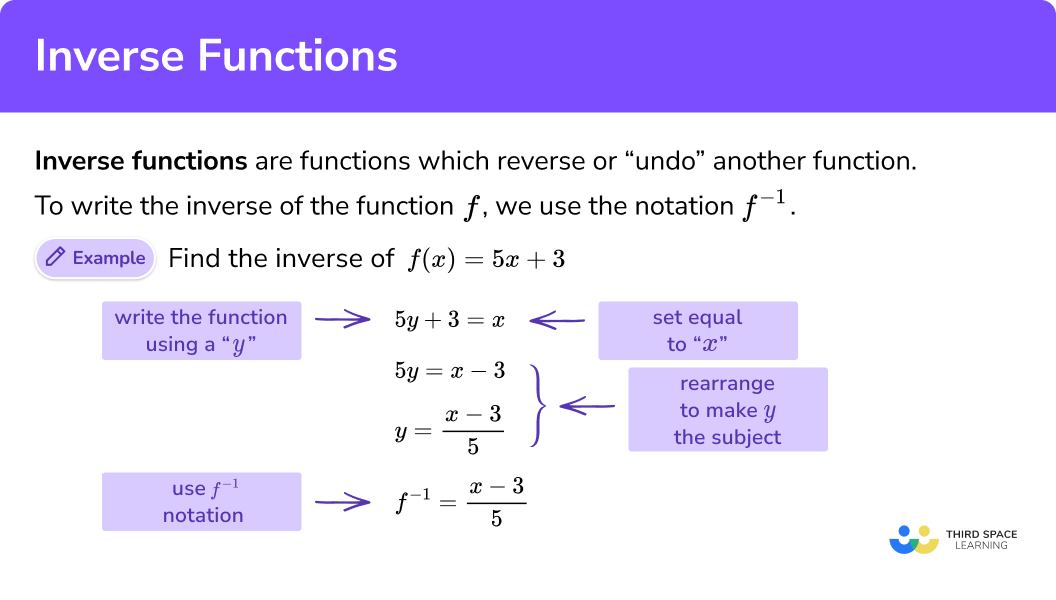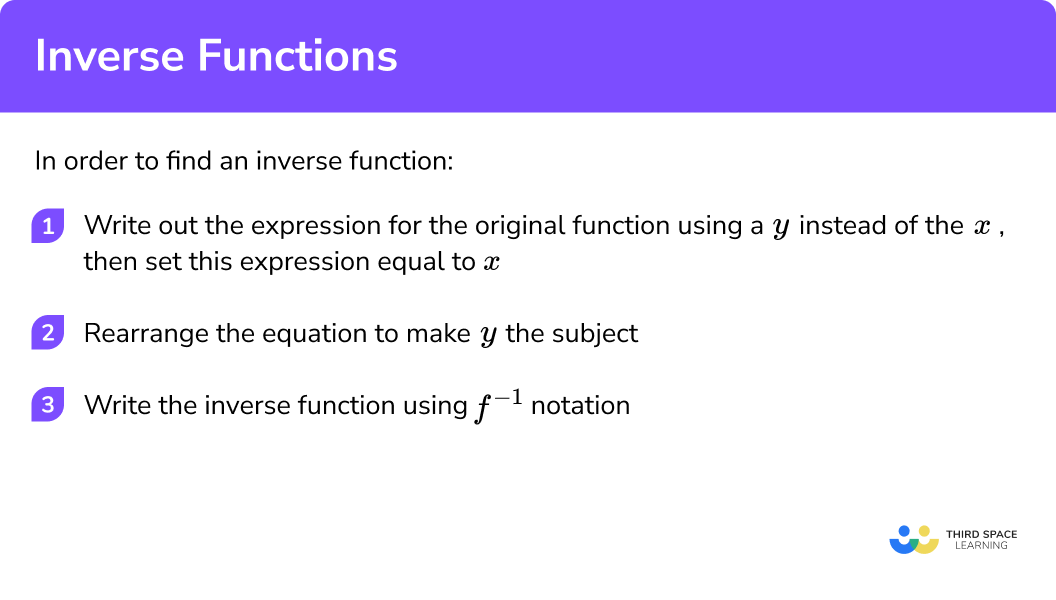GCSE Maths Algebra Functions

Inverse Functions

# Inverse Functions

Here we will learn about inverse functions including what an inverse function is, the notation used for an inverse function and how to find an inverse function.

There are also inverse function worksheets based on Edexcel, AQA and OCR exam questions, along with further guidance on where to go next if you’re still stuck.

## What are inverse functions?

Inverse functions are functions which reverse or “undo” another function.

To write the inverse of the function f , we use the notation f^{-1} .

We have seen how to use a function machine to work backwards to find the input from a known output. If we were to write the algebraic expression relating to these inverse operations in the correct order, we would have found the inverse function.

To find an inverse function we need to rewrite the function using y as the unknown variable and set the function equal to x. Then we need to rearrange the function to make y the subject and write the function using the inverse function notation.

E.g.

Inverse functions can be used to solve equations or find missing x values on graphs if we know the y value.

Inverse functions are also used when finding an unknown angle in a triangle using trigonometry.

E.g.

When finding a missing angle or solving the equation sin (x)=0.6 , we would need to use the inverse of the sine function, x=sin^{-1}(0.6).

In A level mathematics instead of using sin^{-1}, cos^{-1} and tan^{-1}, the inverse trigonometric functions have different names. We would use arcsin, arccos and arctan.

We also look at when inverse functions can exist. Functions such as quadratics, cubics, sin, cos and tan must have a restricted domain (a limit to which x values are allowed).

We also look at how to find the graph of the inverse function f^{-1} by reflecting the graph of the function f in the line y=x .

### What are inverse functions?## How to find inverse functions

In order to find an inverse function:

1. Write out the expression for the original function using a y instead of the x . Set this expression equal to x.
2. Rearrange the equation to make y the subject.
3. Write your inverse function using the f^{-1} notation.

### Explain how to find inverse functions### Related lessons onfunctions in algebra

Inverse functions is part of our series of lessons to support revision on functions in algebra. You may find it helpful to start with the main functions in algebra lesson for a summary of what to expect, or use the step by step guides below for further detail on individual topics. Other lessons in this series include:

## Inverse functions examples

### Example 1: Finding the inverse of a linear function

If f(x)=3x-7 , find f^{-1}(x).

1. Write out the expression for the original function using a y instead of the x . Set this expression equal to x.

3y-7=x

2Rearrange the equation to make y the subject.

\begin{aligned} & 3y-7=x \\\\ & 3y=x+7 \hspace{2cm} \text{Add } 7 \text{ to both sides of the equation} \\\\ & y=\frac{x+7}{3} \hspace{2cm} \text{Divide both sides by } 3 \end{aligned}

3Write your inverse function using the f^{-1} notation.

{{f}^{-1}}\left( x \right)=\frac{x+7}{3}

### Example 2: Finding the inverse of a linear function

If g(x)=9-\frac{x}{2} find g^{-1}(x) .

9-\frac{y}{2}=x

\begin{aligned} & 9-\frac{y}{2}=x \\\\ & 9-x=\frac{y}{2} \hspace{2cm} \text{Add } \frac{y}{2} \text{ to both sides and subtract } x \text{ from both sides. } \\\\ & 18-2x=y \hspace{1.8cm} \text{Multiply both sides by } 2. \end{aligned}

Use g^{-1} because the original function was g.
{{g}^{-1}}\left( x \right)=18-2x

### Example 3: Finding the inverse of a function involving a power

If f(x)=2x^2-5 find f^{-1}(x) .

2{{y}^{2}}-5=x

\begin{aligned} & 2{{y}^{2}}-5=x \\\\ & 2{{y}^{2}}=x+5 \hspace{3cm} \text{Add } 5 \text{ to both sides.. } \\\\ & {{y}^{2}}=\frac{x+5}{2} \hspace{3cm} \text{Divide both sides by } 2. \\\\ & y=\sqrt{\frac{x+5}{2}} \hspace{2.8cm} \text{Square root both sides.} \end{aligned}

An inverse function cannot have two different outputs for one input so we only need the positive square root.

{{f}^{-1}}\left( x \right)=\sqrt{\frac{x+5}{2}}

### Example 4:  Finding the inverse of a function involving an algebraic fraction

If h(x)=\frac{x-3}{x+2} , find h^{-1}(x) .

\frac{y-3}{y+2}=x

\begin{aligned} & \frac{y-3}{y+2}=x \\\\ & y-3=x\left( y+2 \right) \hspace{2.7cm} \text{Multiply both sides by the denominator } y+2 \\\\ & y-3=xy+2x \hspace{2.8cm} \text{Expand the bracket} \\\\ & y-xy=2x+3 \hspace{2.8cm} \text{Isolate the } y \text{ by subtracting } xy \text{ from both sides}\\ & \hspace{5cm} \text{and adding } 3 \text{ to both sides} \\\\ & y\left( 1-x \right)=2x+3 \hspace{2.5cm} \text{Factor out the } y\\\\ & y=\frac{2x+3}{1-x} \hspace{3.4cm} \text{Divide both sides by } 1-x \end{aligned}

{{h}^{-1}}\left( x \right)=\frac{2x+3}{1-x}

### Common misconceptions

• 1 over f(x)

A common error is to think the inverse function will be the reciprocal of the function because of the “power of -1 ” notation.

E.g.
If f(x)=3x, an incorrect expression for f^{-1} would be (3x)^{-1} or \frac{1}{3x} .

### Practice inverse function questions

1. If f(x)=4x-9, find f^{-1}(x):

\frac{x-9}{4}\frac{x+9}{4}\frac{x}{4}+9\frac{x}{4}-9Rearranging the formula, first add 9, then divide by 4.

2. If g(x)=\frac{x}{3}+7, find g^{-1}(x):

3x-213x-73x+213x+7Rearranging the formula, first subtract 7, then multiply by 3.

3. If h(x)=5-x, find h^{-1}(x) :

x-5x+55x5-xRearranging the formula, first add y to both sides, then subtract x from both sides.

4. If f(x)=x^3-1, find f^{-1}(x):

\sqrt{x}+1\sqrt{x+1}x3+1\sqrt{x-1}Rearranging the formula, first add 1, then cube root.

5. If g(x)=\sqrt{x}+6, find g^{-1}(x) :

x^2+36x^2-6x^2-12x+36x^2+6Rearranging the formula, first subtract 6, then square and expand the brackets.

6. If h(x)=\frac{2x}{x+3}, find h^{-1}(x) :

\frac{3x}{2+x}\frac{3x}{x-2}\frac{2x}{x-3}\frac{3x}{2-x}See working and use example 4 to help.

\begin{aligned} & \frac{2y}{y+3}=x \\\\ & 2y=x\left( y+3 \right) \\\\ & 2y=xy+3x \\\\ & 2y-xy=3x \\\\ & y\left( 2-x \right)=3x \\\\ & y=\frac{3x}{2-x} \\\\ & {{h}^{-1}}\left( x \right)=\frac{3x}{2-x} \end{aligned}

### Inverse functions GCSE questions

1. (a) If f(x)=6x-5, find f^{-1}(x).

(b) If g(x)=3+\sqrt{x}, find g^{-1}(x).

(4 marks)

(a)

Attempt the rearrange formula 6y-5=x

(1)

\frac{x+5}{6}

(1)

(b)

Attempt the rearrange formula 3+\sqrt{y}=x

(1)

(x-3)^2   or  x^2-6x+9

(1)

2. If f(x)=3x-2 and g(x)=4x+7

(a) Find f^{-1}(4)

(b) Find when f(x)=g^{-1}(x)

(6 marks)

(a)

Set 3x-2=4 or find f^{-1}(x)=\frac{x+2}{3}

(1)

(1)

(b)

g^{-1}(x)=\frac{x-7}{4}

(1)

Set equal 3x-2=\frac{x-7}{4}

(1)

Get x’s on one side of the equation 12x-x=-7+8

(1)

(1)

3.  If f(x)=\frac{x+5}{x+3}, find f^{-1}(x).

(4 marks)

x=\frac{y+5}{y+3}

x(y+3)=y+5   Multiplying by denominator

(1)

xy-y=5-3x   Expand and isolate

(1)

y(x-1)=5-3x   y factored out

(1)

f^{-1}(x)=\frac{5-3x}{x-1}

(1)

## Learning checklist

You have now learned how to:

• where appropriate, interpret simple expressions as functions with inputs and outputs
• interpret the reverse process as the ‘inverse function’

## Still stuck?

Prepare your KS4 students for maths GCSEs success with Third Space Learning. Weekly online one to one GCSE maths revision lessons delivered by expert maths tutors.

Find out more about our GCSE maths tuition programme.